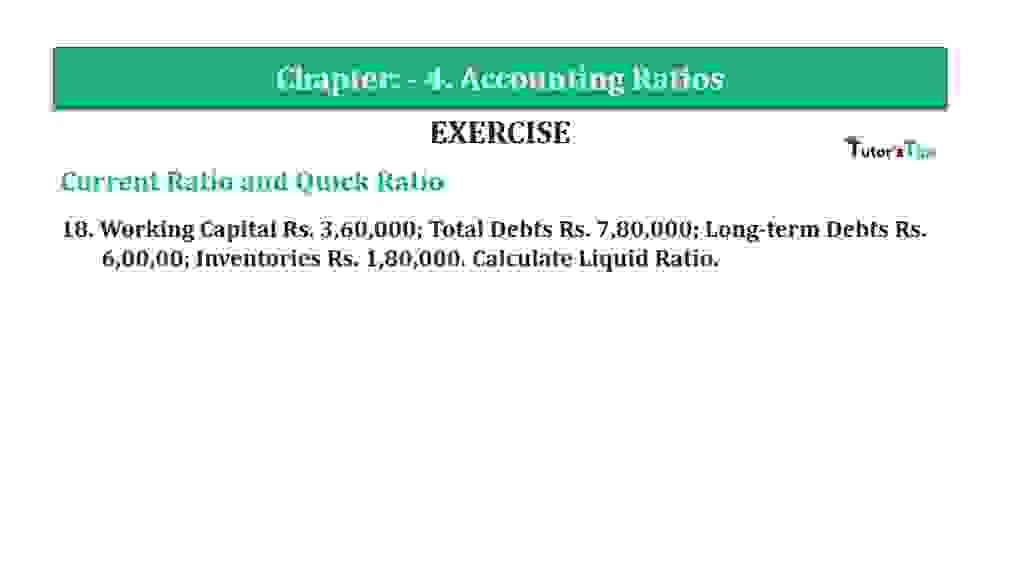# Question 18 Chapter 4 of +2-B – T.S. Grewal 12 ClassQuestion 18 Chapter 4 of +2-B

Current Ratio and Quick Ratio

18. Working Capital Rs. 3,60,000; Total Debts Rs. 7,80,000; Long-term Debts Rs.6,00,00; Inventories Rs. 1,80,000. Calculate Liquid Ratio.

### The solution of Question 18 Chapter 4 of +2-B: –

 Long-term Debts = Rs. 6,00,000 Total Debts = Rs. 7,80,000 Working Capital = Rs. 3,60,000 Inventories = Rs. 1,80,000 Current Liabilities = Total debts – Long-term Debts = Rs. 7,80,000 – Rs.6,00,000 = Rs. 1,80,000

 Current Assets = Current Liabilities + Working Capital = Rs. 1,80,000 + Rs. 3,60,000 Current Assets = Rs. 5,40,000

 Quick Assets = Current Assets – Inventories = Rs. 5,40,000 – Rs. 1,80,000 Current Assets = Rs. 3,60,000

 Current Ratio = Quick Assets Current Liabilities
 = Rs.3,60,000 Rs.1,80,000

Current Ratio= 2 : 1

Balance Sheet: Meaning, Format & Examples

Thanks, Please Like and share with your friends

Comment if you have any question.

Also, Check out the solved question of previous Chapters: –

### T.S. Grewal’s Double Entry Book Keeping (Vol. II: Accounting for Companies)T.S. Grewal’s Analysis of Financial Statements